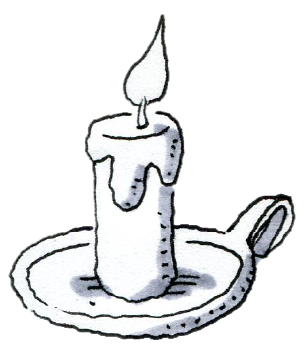### Home > ACC7 > Chapter 8 Unit 9 > Lesson CC3: 8.2.2 > Problem8-67

8-67.

Janet lit a $12$-inch candle. She noticed that it was getting an inch shorter every $30$ minutes.

1. Is the association between the time the candle is lit and the height of the candle positive or negative?

Since the candle is melting, it is getting shorter over time. Think about this on a graph of height vs. time. As the time increases, the height would decrease. What correlation does this represent?

Negative correlation

2. In how many hours will the candle burn out? Support your answer with a reason.• If the candle melts $1$ inch every $30$ minutes, and the candle was originally $12$ inches, how many $30$ minute periods would it take for the candle to melt completely?

• Another way you could look at it is through an algebraic expression: $12 − 2x = 0$
The candle is originally $12$inches, but loses $2$ inches every hour. After how many hours does it melt completely and burn out?

$6$ hours